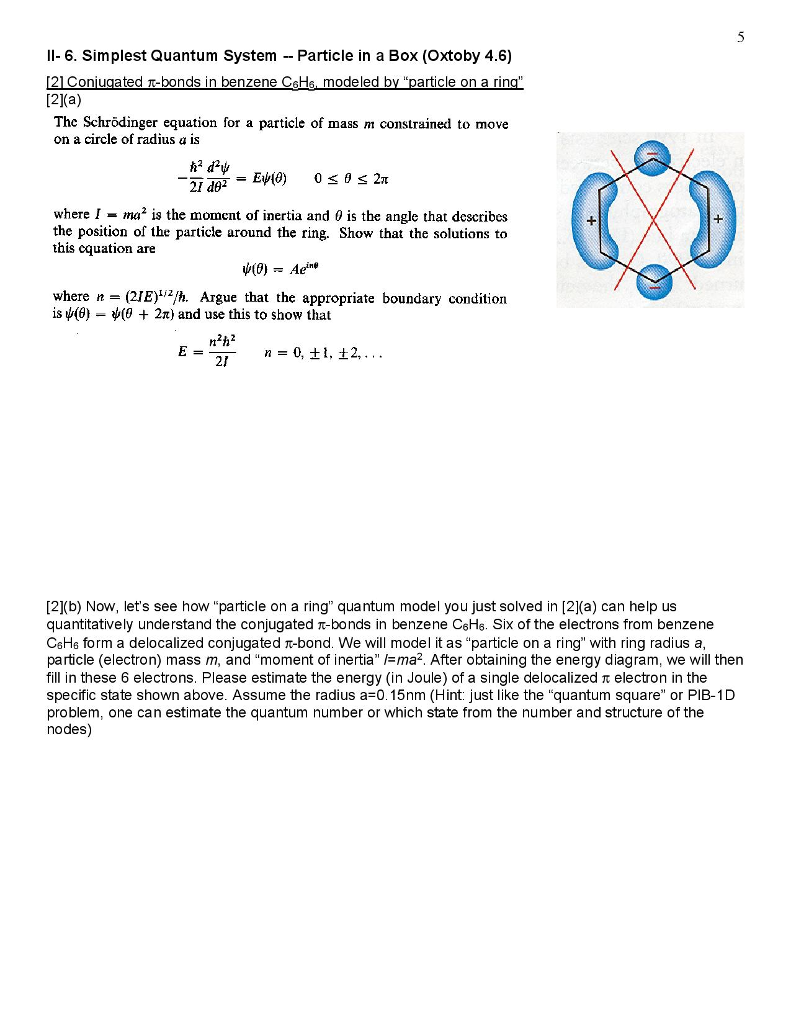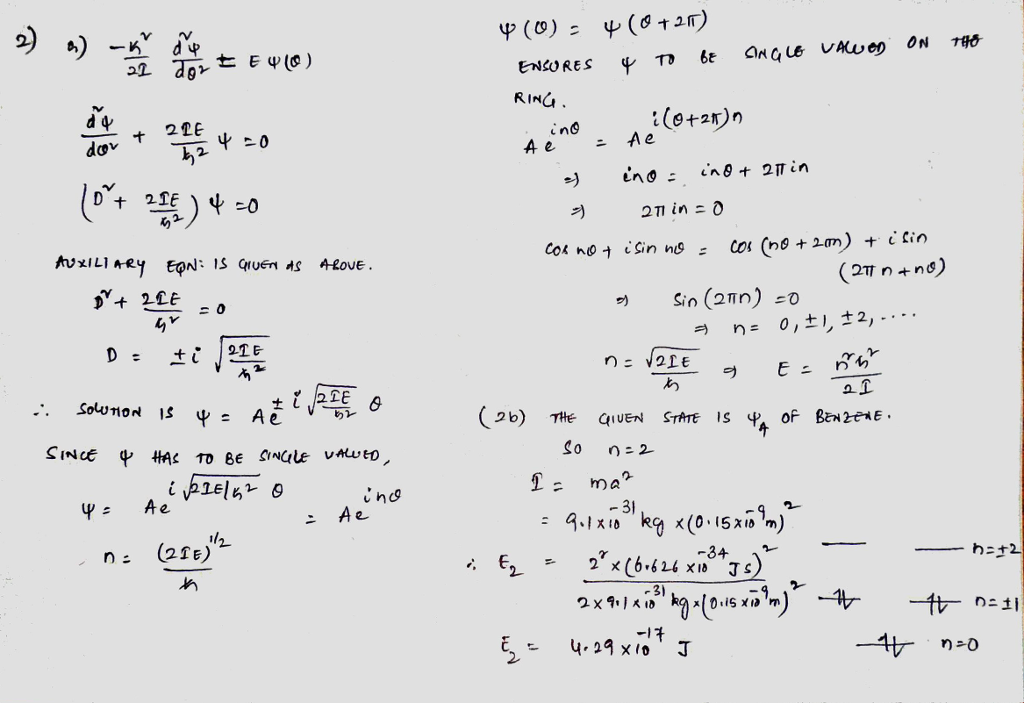# Question & Answer: Il- 6. Simplest Quantum System -Particle in a Box (Oxtoby 4.6) [21(a) The Schrõdinger…..Il- 6. Simplest Quantum System -Particle in a Box (Oxtoby 4.6) [21(a) The Schrõdinger equation for a particle of mass m constrained to move on a circle of radius a is 2I d02 where I ma2 is the moment of inertia and 0 is the angle that describes the position of the particle around the ring. Show that the solutions to this cquation are where n= (21E)112/h. Argue that the appropriate boundary condition is ψ(θ)-ψ(9 + 2π) and use this to show that =21- n-0, tl, ±2,..。 [2(b) Now, let’s see how “particle on a ring” quantum model you just solved in (a) can help us quantitatively understand the conjugated π-bonds in benzene C6H6. Six of the electrons from benzene C6H6 form a delocalized conjugated π-bond. We will model it as ‘particle on a ring” with ring radius a particle (electron) mass m, and “moment of inertia” ma-. After obtaining the energy diagram, we will then fill in these 6 electrons. Please estimate the energy (in Joule) of a single delocalized π electron in the specific state shown above. Assume the radius a=0.15nm (Hint just like the “quantum square” or PIB-1D problem, one can estimate the quantum number or which state from the number and structure of the nodes)